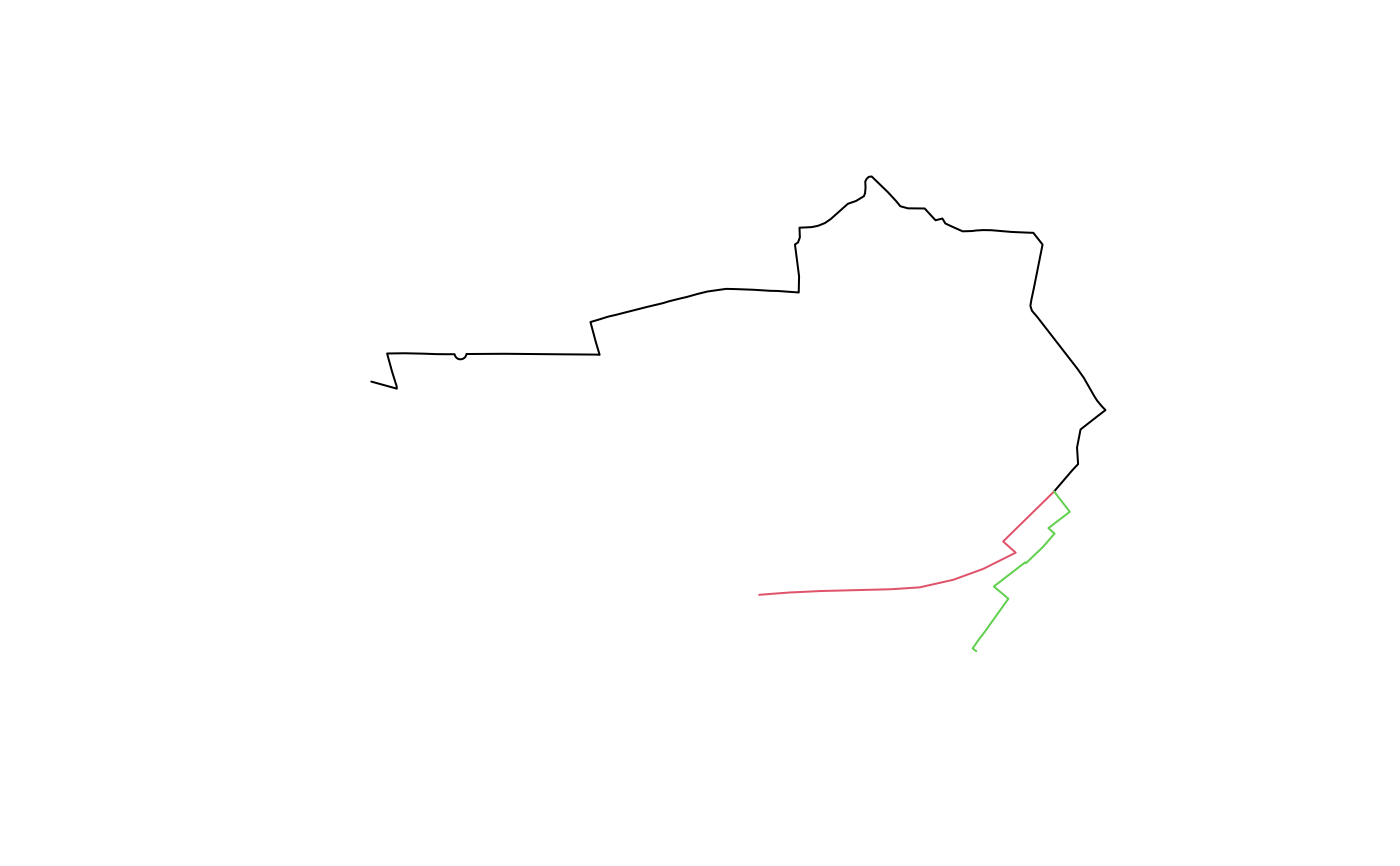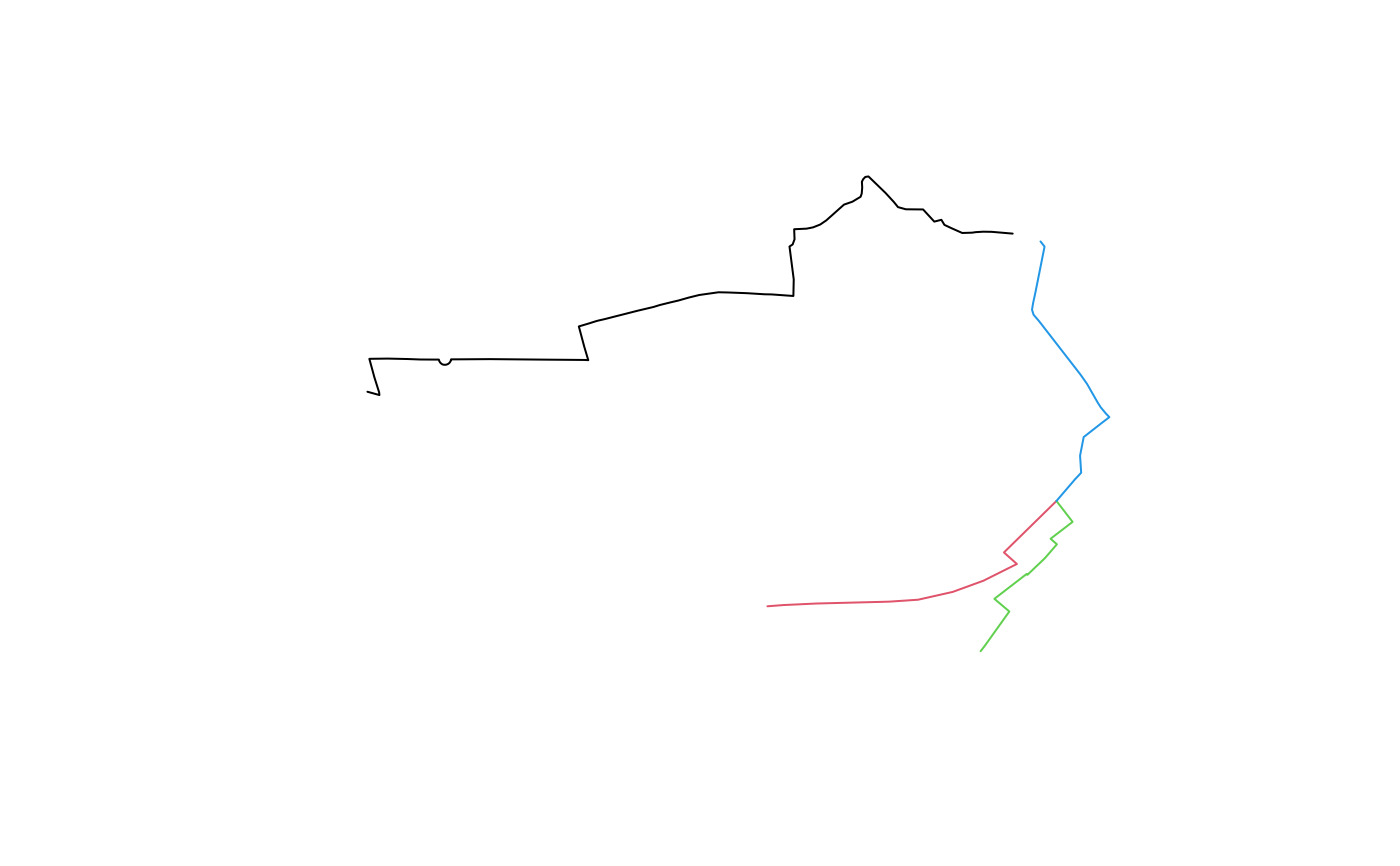Divides SpatialLinesDataFrame objects into separate Lines. Each new Lines object is the aggregate of a single number of aggregated lines.

## Usage

``gsection(sl, buff_dist = 0)``

## Arguments

sl

SpatialLinesDataFrame with overlapping Lines to split by number of overlapping features.

buff_dist

A number specifying the distance in meters of the buffer to be used to crop lines before running the operation. If the distance is zero (the default) touching but non-overlapping lines may be aggregated.

Other rnet: `islines()`, `overline()`, `rnet_breakup_vertices()`, `rnet_group()`

## Examples

``````lib_versions <- sf::sf_extSoftVersion()
lib_versions
#>           GEOS           GDAL         proj.4 GDAL_with_GEOS     USE_PROJ_H
#>       "3.10.2"        "3.4.1"        "8.2.1"         "true"         "true"
#>           PROJ
#>        "8.2.1"
# fails on some systems (with early versions of PROJ)
if (lib_versions >= "6.3.1") {
sl <- routes_fast_sf[2:4, ]
rsec <- gsection(sl)
length(rsec) # sections
plot(rsec, col = seq(length(rsec)))
rsec <- gsection(sl, buff_dist = 50)
length(rsec) # 4 features: issue
plot(rsec, col = seq(length(rsec)))
}``````